# help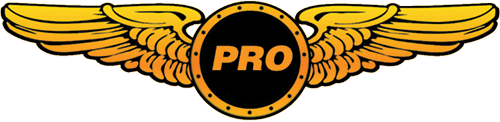Ben (eagle3) First Officer

hi i aksidently messed up my c182 cfg. file and i was wondering if yuo know of any way to get it back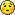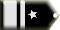tomthetank Chief Captain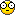What do you mean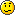messed up

No matter,copy and paste this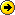----------------------------------------------------------------------

[fltsim.0]
title=Cessna Skylane 182S
sim=Cessna182S
model=
panel=
sound=
texture=
kb_checklists=Cessna182S_check
kb_reference=Cessna182S_ref
atc_id=N778WS
ui_manufacturer=Cessna
ui_type="C182S Skylane"
ui_variation="White with green"
description="When Cessna saw how well their Model 180 was selling, they looked for a way to make it an even bigger success\s the answer was the Model 182. The Cessna 182 Skylane feels and acts like a heavier, more powerful version of its sibling, the 172 Skyhawk. The airplane is a workhorse and a stable platform for flying on instruments. Even with a full tank, the 182 carries a family-size useful load and performs admirably as an aerial sport utility vehicle."

[fltsim.1]
title=Cessna Skylane 182S IFR
sim=Cessna182S
model=
panel=ifr
sound=
texture=
kb_checklists=Cessna182S_check
kb_reference=Cessna182S_ref
atc_id=N267QD
ui_manufacturer=Cessna
ui_type="C182S Skylane"
ui_variation="White with green - IFR panel"
description="When Cessna saw how well their Model 180 was selling, they looked for a way to make it an even bigger success\s the answer was the Model 182. The Cessna 182 Skylane feels and acts like a heavier, more powerful version of its sibling, the 172 Skyhawk. The airplane is a workhorse and a stable platform for flying on instruments. Even with a full tank, the 182 carries a family-size useful load and performs admirably as an aerial sport utility vehicle."

[fltsim.2]
title=Cessna Skylane 182S Paint1
sim=Cessna182S
model=
panel=
sound=
texture=1
kb_checklists=Cessna182S_check
kb_reference=Cessna182S_ref
atc_id=N549YB
ui_manufacturer=Cessna
ui_type="C182S Skylane"
ui_variation="White with red and yellow"
description="When Cessna saw how well their Model 180 was selling, they looked for a way to make it an even bigger success\s the answer was the Model 182. The Cessna 182 Skylane feels and acts like a heavier, more powerful version of its sibling, the 172 Skyhawk. The airplane is a workhorse and a stable platform for flying on instruments. Even with a full tank, the 182 carries a family-size useful load and performs admirably as an aerial sport utility vehicle."

[fltsim.3]
title=Cessna Skylane 182S Paint2
sim=Cessna182S
model=
panel=
sound=
texture=2
kb_checklists=Cessna182S_check
kb_reference=Cessna182S_ref
atc_id=N9COF
ui_manufacturer=Cessna
ui_type="C182S Skylane"
ui_variation="White with blue and green"
description="When Cessna saw how well their Model 180 was selling, they looked for a way to make it an even bigger success\s the answer was the Model 182. The Cessna 182 Skylane feels and acts like a heavier, more powerful version of its sibling, the 172 Skyhawk. The airplane is a workhorse and a stable platform for flying on instruments. Even with a full tank, the 182 carries a family-size useful load and performs admirably as an aerial sport utility vehicle."

[fltsim.4]
title=Cessna Skylane 182S Paint3
sim=Cessna182S
model=
panel=
sound=
texture=3
kb_checklists=Cessna182S_check
kb_reference=Cessna182S_ref
atc_id=N882LS
ui_manufacturer=Cessna
ui_type="C182S Skylane"
ui_variation="White with blue and purple"
description="When Cessna saw how well their Model 180 was selling, they looked for a way to make it an even bigger success\s the answer was the Model 182. The Cessna 182 Skylane feels and acts like a heavier, more powerful version of its sibling, the 172 Skyhawk. The airplane is a workhorse and a stable platform for flying on instruments. Even with a full tank, the 182 carries a family-size useful load and performs admirably as an aerial sport utility vehicle."

[General]
atc_type=Cessna
atc_model=C182
editable=0
performance="Maximum Speed\t\n145 kts 167 mph\t269 km\/h\n\nCruise Speed\t\n140 kts 161 mph \t259 km\/h\n\nEngine\t\nTextron Lycoming IO-540-AB1A5 230 hp\n\nPropeller\t\nThree-bladed McCauley constant speed\n\nMaximum Range\t\n968 nm 1,114 sm \t1,793 km\n\nService Ceiling\t\n18,100 ft \t 5,517 m\n\nFuel Capacity\t\n92 U.S. gal 348 L \n\nEmpty Weight\t\n1,810 lb \t 821 kg\n\nMaximum Gross Weight\t\n3,110 lb \t 1,411 kg\n\nLength\t\n29 ft\t 8.84 m\n\nWingspan\t\n36 ft\t 11 m\n\nHeight\t\n9 ft\t 2.77 m\n\nSeating\t\nUp to 4 \n\nUseful Load\t\n1,300 lb \t 590 kg"

[WEIGHT_AND_BALANCE]
max_gross_weight = 3110 // (pounds)
empty_weight = 1810 // (pounds)

reference_datum_position = 3.6, 0, 0 // (feet) distance from FlightSim Reference position: (1/4 chord, centerline, waterline)
empty_weight_CG_position = -3.0, 0, 0 // (feet) longitudinal, lateral, vertical distance from specified datum

max_number_of_stations = 50

station_load.0 = "170, -3.0, -1.5, 0.0, Pilot" // Weight (lbs), longitudinal, lateral, vertical positions from datum (feet)
station_load.1 = "170, -3.0, 1.5, 0.0, Front Passenger" // Weight (lbs), longitudinal, lateral, vertical positions from datum (feet)
station_load.2 = "160, -6.2, -1.5, 0.0, Rear Passenger" // Weight (lbs), longitudinal, lateral, vertical positions from datum (feet)
station_load.3 = "160, -6.2, 1.5, 0.0, Rear Passenger" // Weight (lbs), longitudinal, lateral, vertical positions from datum (feet)
station_load.4 = "60, -8.0, 0.0, 0.0, Baggage" // Weight (lbs), longitudinal, lateral, vertical positions from datum (feet)

;Moments of Inertia
empty_weight_pitch_MOI = 1400.0
empty_weight_roll_MOI = 1137.0
empty_weight_yaw_MOI = 2360.0
empty_weight_coupled_MOI= 0.0

[flight_tuning]
cruise_lift_scalar = 1.0
parasite_drag_scalar = 1.0
induced_drag_scalar = 1.0
elevator_effectiveness = 1.0
aileron_effectiveness = 1.0
rudder_effectiveness = 1.0
pitch_stability = 1.0
roll_stability = 1.0
yaw_stability = 1.0
elevator_trim_effectiveness = 1.0
aileron_trim_effectiveness = 1.0
rudder_trim_effectiveness = 1.0

[GeneralEngineData]
engine_type = 0 //0=Piston, 1=Jet, 2=None, 3=Helo-Turbine, 4=Rocket, 5=Turboprop
Engine.0 = 2.4, 0.0, 0.0 //(feet) longitudinal, lateral, vertical distance from reference datum
fuel_flow_scalar= 1.0 //Fuel flow scalar
min_throttle_limit = 0.0 //Minimum percent throttle. Generally negative for turbine reverser

[piston_engine]
power_scalar = 1.0 //Piston power scalar
cylinder_displacement= 90.3 //Cubic inches per cylinder
compression_ratio= 8.5 //Compression ratio
number_of_cylinders=6 //Number of cylinders
max_rated_rpm= 2400 //Max rated RPM
max_rated_hp= 230 //Max rated HP
fuel_metering_type= 0 //0=Fuel Injected, 1=Gravity Carburetor, 2=Aerobatic Carburetor
cooling_type= 0 //0=Cooling type Air, 1=Cooling type Liquid
normalized_starter_torque= 0.3 //Starter torque factor
turbocharged= 0 //Is it turbocharged? 0=FALSE, 1=TRUE
max_design_mp= 0 //Max design manifold pressure, (inHg)
min_design_mp= 0 //Min design manifold pressure, (inHg)
critical_altitude= 0 //Altitude to which the turbocharger will provide max design manifold pressure (feet)
emergency_boost_type= 0 //0=None, 1=Water Injection, 2=Methanol/Water injection, 3=War Emergency Power
emergency_boost_mp_offset= 0 //Additional manifold pressure supplied by emergency boost
emergency_boost_gain_offset= 0 //Multiplier on manifold pressure due to emergency boost
fuel_air_auto_mixture= 0 //Automixture available? 0=FALSE, 1=TRUE
auto_ignition= 0 //Auto-Ignition available? 0=FALSE, 1=TRUE
max_rpm_mechanical_efficiency_scalar= 1.0 //Scalar on maximum RPM mechanical efficiency
idle_rpm_mechanical_efficiency_scalar= 1.0 //Scalar on idle RPM mechanical efficiency
max_rpm_friction_scalar= 1.0 //Scalar on maximum RPM friction
idle_rpm_friction_scalar= 1.0 //Scalar on idle RPM friction

[propeller]
thrust_scalar = 1.0 //Propeller thrust scalar
propeller_type= 0 //0=Constant Speed, 1=Fixed Pitch
propeller_diameter= 6.58 //Propeller Diameter, (feet)
propeller_moi= 8.3 //Propeller moment of inertia
beta_max= 31.7 //Maximum blade pitch angle for constant speed prop, (degrees)
beta_min= 14.9 //Minimum blade pitch angle for constant speed prop, (degrees)
min_gov_rpm= 1400 //Miminum governed RPM
prop_tc= 0.1 //Prop time-constant
gear_reduction_ratio= 1.0 //Propeller gear reduction ratio
fixed_pitch_beta= 20 //Fixed pitch angle of fixed pitch prop, (degrees)
low_speed_theory_limit= 80 //Speed at which low speed theory becomes blended in (feet/second)
prop_sync_available= 0 //Prop synchronization available? 0=FALSE, 1=TRUE
prop_deice_available= 0 //Prop de-icing available? 0=FALSE, 1=TRUE
prop_feathering_available= 0 //Prop feathering available? 0=FALSE, 1=TRUE
prop_auto_feathering_available= 0 //Prop auto-feathering available? 0=FALSE, 1=TRUE
min_rpm_for_feather= 0 //Minimum RPM for prop feathering
beta_feather= 0 //Feathering pitch angle (degrees)
power_absorbed_cf= 0 //Coefficient of friction for power absorbed by propeller
defeathering_accumulators_available= 0 //Defeathering accumulators available? 0=FALSE, 1=TRUE
prop_reverse_available= 0 //Prop reverse available? 0=FALSE, 1=TRUE
minimum_on_ground_beta= 0 //Miminum pitch angle on ground, (degrees)
minimum_reverse_beta= 0 //Minimum pitch angle in reverse, (degrees)

[electrical]
flap_motor = 0, 5 , 17.0
gear_motor = 0, 5 , 17.0
autopilot = 0, 5 , 17.0
avionics_bus = 0, 5 , 17.0
avionics = 1, 5 , 17.0
pitot_heat = 0, 2 , 17.0
additional_system = 0, 2 , 17.0
marker_beacon = 1, 2 , 17.0
gear_warning = 0, 2 , 17.0
fuel_pump = 0, 5 , 17.0
starter1 = 0, 20, 17.0
light_nav = 0, 5 , 17.0
light_beacon = 0, 5 , 17.0
light_landing = 0, 5 , 17.0
light_taxi = 0, 5 , 17.0
light_strobe = 0, 5 , 17.0
light_panel = 0, 5 , 17.0

//0 Class
//1 Longitudinal Position (feet)
//2 Lateral Position (feet)
//3 Vertical Position (feet)
//4 Impact Damage Threshold (Feet Per Minute)
//5 Brake Map (0=None, 1=Left, 2=Right)
//7 Steer Angle (degrees)
//8 Static Compression (feet) (0 if rigid)
//9 Max/Static Compression Ratio
//10 Damping Ratio (0=Undamped, 1=Critically Damped)
//11 Extension Time (seconds)
//12 Retraction Time (seconds)
//13 Sound Type
[contact_points]
point.0 = 1, 0.48, 0.00, -3.58, 1500, 0, 0.5, 22.0, 0.3, 2.5, 0.7, 0.0, 0.0, 0
point.1 = 1, -5.02, -4.50, -3.33, 2500, 1, 0.5, 0.0, 0.3, 2.5, 0.7, 0.0, 0.0, 2
point.2 = 1, -5.02, 4.50, -3.33, 2500, 2, 0.5, 0.0, 0.3, 2.5, 0.7, 0.0, 0.0, 3
point.3 = 2, -3.60, -17.9, 2.08, 1574.8, 0, 0.000, 0.0, 0.0, 0.0, 0.0, 0.0, 0.0, 5, 0.0, 0.0
point.4 = 2, -3.60, 17.9, 2.08, 1574.8, 0, 0.000, 0.0, 0.0, 0.0, 0.0, 0.0, 0.0, 6, 0.0, 0.0
point.5 = 2, -19.93, 0.0, 0.83, 1574.8, 0, 0.000, 0.0, 0.0, 0.0, 0.0, 0.0, 0.0, 9, 0.0, 0.0
point.6 = 2, 1.40, 0.0, -1.33, 1574.8, 0, 0.000, 0.0, 0.0, 0.0, 0.0, 0.0, 0.0, 4, 0.0, 0.0
point.7 = 2, -19.93, 0.0, 6.83, 1574.8, 0, 0.000, 0.0, 0.0, 0.0, 0.0, 0.0, 0.0, 7, 0.0, 0.0
point.8 = 2, -4.60, 0.0, -1.33, 1574.8, 0, 0.000, 0.0, 0.0, 0.0, 0.0, 0.0, 0.0, 8, 0.0, 0.0

max_number_of_points = 21

static_pitch=3.82 //degrees, pitch when at rest on the ground (+=Up, -=Dn)
static_cg_height=3.015 //feet, altitude of CG when at rest on the ground

[brakes]
parking_brake = 1 //Parking brake available
toe_brakes_scale = 0.68 //Brake scalar

[hydraulic_system]
normal_pressure = 0.0 //PSI

[fuel]
//Tanks Position and capacity:
//Longitudinal (feet), Lateral (feet), Vertical (feet), Usable(gallons), Unusable (gallons)
LeftMain = -3.85, -2.10, 2.50, 46.0, 0.00
RightMain= -3.85, 2.10, 2.50, 46.0, 0.00
//Fuel type: 1 = Avgas, 2 = JetA
fuel_type=1
number_of_tank_selectors = 1
electric_pump=1

[Views]
eyepoint= -3.95, -0.85, 2.1 //(feet) longitudinal, lateral, vertical distance from reference datum

[flaps.0]
type = 1 // 1 - tail, 2 - lead
span-outboard = 0.5 // 0.0 .. 1.0
extending-time = 5 // seconds
flaps-position.0 = 0 // degrees
flaps-position.1 = 10 // degrees
flaps-position.2 = 20 // degrees
flaps-position.3 = 30 // degrees
damaging-speed = 250 // KIAS
blowout-speed = 300 // KIAS
lift_scalar = 1.0
drag_scalar = 1.0
pitch_scalar= 1.0
system_type = 0 //0=Electric, 1=Hydraulic, 2=Pneumatic, 3=Manual, 4=None

// Radio Type = availiable, standby frequency, has glide slope
Audio.1 = 1
Com.1 = 1, 1
Com.2 = 1, 1
Nav.1 = 1, 1, 1
Nav.2 = 1, 1, 0
Transponder.1 = 1
Marker.1 = 1

[keyboard_response]
//Breakpoint speeds (knots) on the keyboard increments of control surfaces.
//Speed at which the incremenet is reduced to 1/2 and 1/8 respectively.
elevator = 100, 180
aileron = 200, 1000
rudder = 200, 1000

[direction_indicators]
//1 Type: 0=None, 1=Vacuum Gyro, 2=Electric Gyro, 3=Electro-Mag Slaved Compass, 4=Slaved to another
//2 Indicator number of slaving indicator if Type = 4
direction_indicator.0=1,0

[attitude_indicators]
//Type: 0=None, 1=Vacuum Gyro, 2=Electric Gyro
attitude_indicator.0 = 1

[turn_indicators]
//Type: 0=None, 1=Electric Gyro, 2=Vacuum Gyro
turn_indicator.0=1,1 //Turn AND Bank

[airspeed_indicators]
airspeed_indicator.0 = 1.3, -24.0

[LIGHTS]
light.0 = 3, -3.03, -18.11, 3.58, fx_navred
light.1 = 3, -3.03, 18.11, 3.58, fx_navgre
light.2 = 3, -21.45, 0.00, 1.95, fx_navwhi
light.3 = 2, -3.33, -18.31, 3.58, fx_strobe
light.4 = 2, -3.33, 18.31, 3.58, fx_strobe
light.5 = 1, -21.52, 0.00, 7.40, fx_beacon
light.6 = 4, -2.24, 0.00, 2.20, fx_vclight

[EFFECTS]
wake=fx_wake
water=fx_spray
dirt=fx_tchdrt
concrete=fx_sparks
touchdown=fx_tchdwn_s, 1

[airplane_geometry]
wing_area = 176.0 //Square feet
wing_span = 36.0 //Feet
wing_root_chord = 4.9 //Feet
wing_dihedral = 1.7 //Degrees
wing_incidence = 1.5 //Degrees
wing_twist = -3.0 //Degrees
oswald_efficiency_factor= 0.7 //Measure of lift effeciency of wing
wing_winglets_flag = 0 //Are winglets available?
wing_sweep = 0.0 //Degrees, wing leading edge
wing_pos_apex_lon = -2.4 //Feet, longitudinal distance from reference point, negative going aft
wing_pos_apex_vert = 0.0 //Feet, vertical distance from reference point, positive going up
htail_area = 39.0 //Square feet
htail_span = 11.7 //Feet
htail_pos_lon = -18.3 //Feet, longitudinal distance from reference point, negative going aft
htail_pos_vert = 0.0 //Feet, vertical distance from reference point, positive going up
htail_incidence = 3.2 //Degrees
htail_sweep = 10.0 //Degrees, horizontal tail leading edge
vtail_area = 18.0 //Square feet
vtail_span = 4.8 //Feet, tip to body
vtail_sweep = 40.0 //Degrees, vertical tail leading edge
vtail_pos_lon = -16.2 //Feet, longitudinal distance from reference point, negative going aft
vtail_pos_vert = 1.5 //Feet, vertical distance from reference point, positive going up
elevator_area = 16.6 //Square feet
aileron_area = 18.3 //Square feet
rudder_area = 6.7 //Square feet
elevator_up_limit = 28.0 //Degrees
elevator_down_limit = 21.0 //Degrees
aileron_up_limit = 20.0 //Degrees
aileron_down_limit = 15.0 //Degrees
rudder_limit = 24.0 //Degrees
elevator_trim_limit = 19.5 //Degrees
spoiler_limit = 0.0 //Degrees
spoilerons_available = 0 //Spoilerons Available?
aileron_to_spoileron_gain = 0 //Aileron to spoileron gain
min_ailerons_for_spoilerons = 0 //Degrees
min_flaps_for_spoilerons = 0 //Minimum flap handle position when spoilerons activate

[Reference Speeds]
flaps_up_stall_speed = 54.0 //Knots True (KTAS)
full_flaps_stall_speed = 49.0 //Knots True (KTAS)
cruise_speed = 120.0 //Knots True (KTAS)
max_indicated_speed = 175 //Red line (KIAS)

[autopilot]
autopilot_available= 1
flight_director_available= 0
default_vertical_speed= 700.0
autothrottle_available= 0
pitch_takeoff_ga=8.0
max_pitch=10.0
max_pitch_acceleration=1.0
max_pitch_velocity_lo_alt=2.0
max_pitch_velocity_hi_alt=1.5
max_pitch_velocity_lo_alt_breakpoint=20000.0
max_pitch_velocity_hi_alt_breakpoint=28000.0
max_bank=25.0
max_bank_acceleration=1.8
max_bank_velocity=3.00
max_throttle_rate=0.10
nav_proportional_control=9.00
nav_integrator_control=0.25
nav_derivative_control=0.00
nav_integrator_boundary=2.50
nav_derivative_boundary=0.00
gs_proportional_control=9.52
gs_integrator_control=0.26
gs_derivative_control=0.00
gs_integrator_boundary=0.70
gs_derivative_boundary=0.00
yaw_damper_gain = 0.0

[forcefeedback]
gear_bump_nose_magnitude=6000 ; 0 - 10000
gear_bump_nose_direction=18000 ; 0 - 35999 degrees
gear_bump_nose_duration=250000 ; in microseconds
gear_bump_left_magnitude=6000 ; 0 - 10000
gear_bump_left_direction=35500 ; 0 - 35999 degrees
gear_bump_left_duration=250000 ; in microseconds
gear_bump_right_magnitude=6000 ; 0 - 10000
gear_bump_right_direction=00500 ; 0 - 35999 degrees
gear_bump_right_duration=250000 ; in microseconds
ground_bumps_magnitude1=3250 ; 0 - 10000
ground_bumps_angle1=8900 ; 0 - 35999 degrees
ground_bumps_intercept1=5.0
ground_bumps_slope1=0.48
ground_bumps_magnitude2=750 ; 0 - 10000
ground_bumps_angle2=9100 ; 0 - 35999 degrees
ground_bumps_intercept2=0.075
ground_bumps_slope2=1.0
crash_magnitude1=10000 ; 0 - 10000
crash_direction1=01000 ; 0 - 35999 degrees
crash_magnitude2=10000 ; 0 - 10000
crash_direction2=9000 ; 0 - 35999 degrees
crash_period2=75000 ; in microseconds
crash_duration2=2500000 ; in microseconds

[stall_warning]
type=2ste (tazz126) First Officer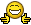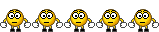good on ttt i bet that took some time welldone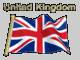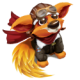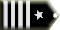Taylor (Flyboy92) Captain

tazz126 wrote:good on ttt i bet that took some time welldone

I bet he just copy/pasted it into the forum. there is NO WAY he could have typed all of that without going mad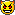. And if he made a typo the WHOLE thing wouldn't work!

Flyboy92Ben (eagle3) First Officer

thankstomthetank Chief Captain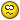Ive been mad for yearsBut,yes I just copy and paste here from the aircraft folder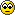ste (tazz126) First Officer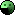hahahaha u smart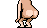u hehehe very clever TTT:D

Last edited by ste (tazz126) on Mon Apr 03, 2006 12:04 am, edited 1 time in totalBy the way i am in R.C.A.F Cadets my rank there is Corporal ... lol just saying.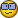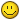ste (tazz126) First Officer

sorry

Last edited by ste (tazz126) on Mon Apr 03, 2006 12:05 am, edited 1 time in totalste (tazz126) First Officer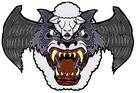Last edited by ste (tazz126) on Mon Apr 03, 2006 12:09 am, edited 1 time in totalste (tazz126) First Officer

I'm a Ci instructor in the atc were im gowning for a commission to wing commander just saying sorry for that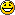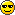All times are GMT Page 1 of 1